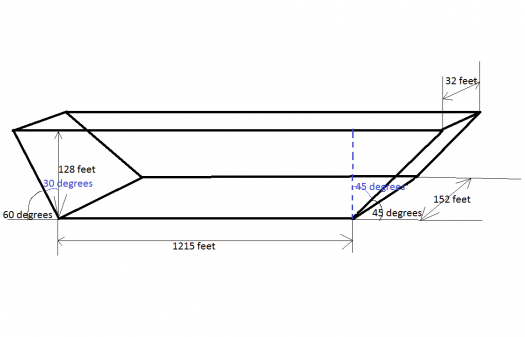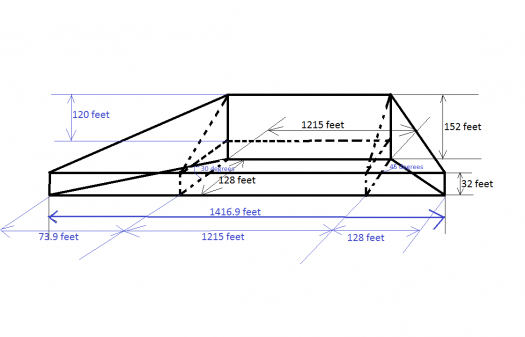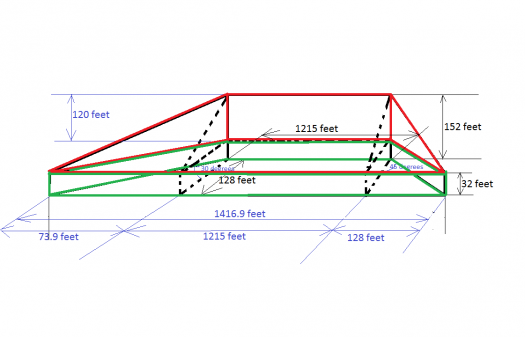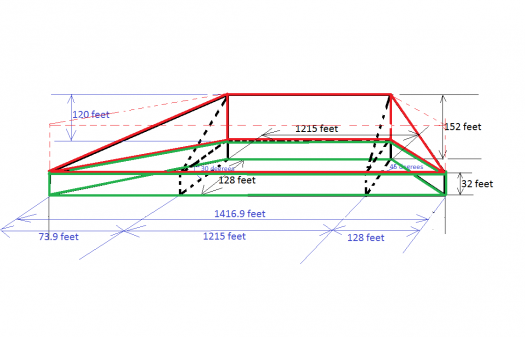# solid geometry

2 posts / 0 new
Renz Kolin Gaba...solid geometry

A concrete dam of hieght 128 ft. was built in a gorge. One side AB of the gorge slopes at an angle of 60 degrees, the other side CD at 45 degrees. The bases of the dam are horizontal and rectangular in shape. The lower base is 1215 ft. by 152 ft., and the upper base is 32 ft. wide. How many cubic yards of concrete were required?

fitzmerl duronTo answer the question above, we need some figure...hehehe...

I imagine that dam to look like this:Delving deeper into the picture, it becomes like this:Positioning the dam so it is easier to get the volume of this dam, it looks like this:Notice that the it is easier to get the volume of the dam if we separate the shapes that makes up the dam, the red and the green part...The green part's volume is pretty straightforward. We'll just use the formula $$V = BH$$, where $V$ is the volume of the figure, $B$ is the area of the base and $H$ is the height of the figure. Looking at the green part of the figure, the area of it's base is a trapezoid. The area would be $$A = \frac{1}{2}h(b_1 + b_2)$$, where $h$ is the height of plane, $b_1$ is the length of the upper base, and $b_2$ is the length of the lower base. With that in mind, the volume of the green part would be...

$$V=BH$$ $$V = \left( \frac{1}{2}h(b_1 + b_2)\right)(H)$$ $$V = \left( \frac{1}{2}(128 \space feet)(1215 \space feet + 1416.9 \space feet)\right)(32 \space feet)$$ $$V = 5390131.2 \space ft^3$$

The red part is pretty tricky, but solvable. Imagining the red part as a one part cut in halves, it becomes easier to get its volume. Getting the volume of the whole red part below:$$V=BH$$ $$V = \left( \frac{1}{2}h(b_1 + b_2)\right)(H)$$ $$V = \left( \frac{1}{2}(128 \space feet)(1215 \space feet + 1416.9 \space feet)\right)(120 \space feet)$$ $$V = 20212992 \space ft^3$$

Now getting the volume of the red part we see, it is $\frac{V}{2}$ or $10106496\space ft^3$

The total volume of the dam would be $5390131.2 \space ft^3 + 10106496 \space ft^3$ or $15496627 \space ft^3$

It's not yet the final answer because it must be converted to cubic yards. Now converting from cubic feet to cubic yards:

$$15496627 \space ft^3\left(\frac{1 \space meters}{3.28 \space feet}\right)^3 = 439152.79 \space m^3$$ $$439152.79 \space m^3 \left( \frac{1 \space yards}{0.9144 \space meter}\right)^3 = \color{green}{574390.16 \space cubic \space yards}$$

Alternate solutions are highly encouraged......

Subscribe to MATHalino on